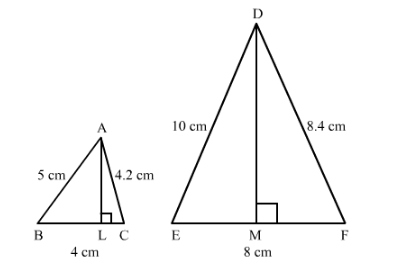# In ∆ABC and ∆DEF, it is being given that: AB = 5 cm, BC = 4 cm and CA = 4.2 cm;

Question:

In ∆ABC and ∆DEF, it is being given that: AB = 5 cm, BC = 4 cm and CA = 4.2 cm; DE = 10 cm, EF = 8 cm and FD = 8.4 cm. If AL ⊥ BC and DM ⊥ EF, find AL : DM.

Solution:

It is given that $A B=5 \mathrm{~cm}, B C=4 \mathrm{~cm}, C A=4.2 \mathrm{~cm}, D E=10 \mathrm{~cm}, E F=8 \mathrm{~cm}$ and $F D=8.4 \mathrm{~cm}$.We have to find $A L: D M$

Since both triangle are similar

So, $\frac{A B}{D E}=\frac{B C}{E F}=\frac{A C}{D F}=\frac{1}{2}$

Here, we use the result that in similar triangle the ratio of corresponding altitude is same as the ratio of the corresponding sides.

$\therefore A L: D M=1: 2$